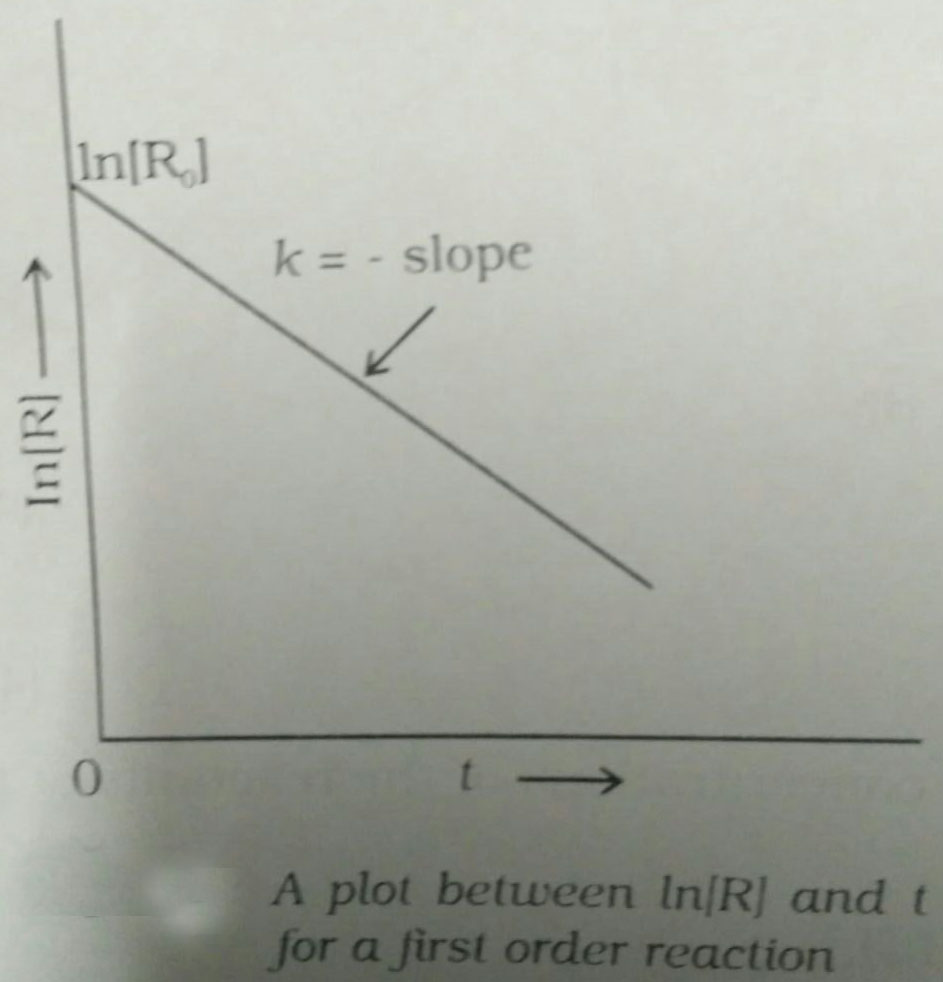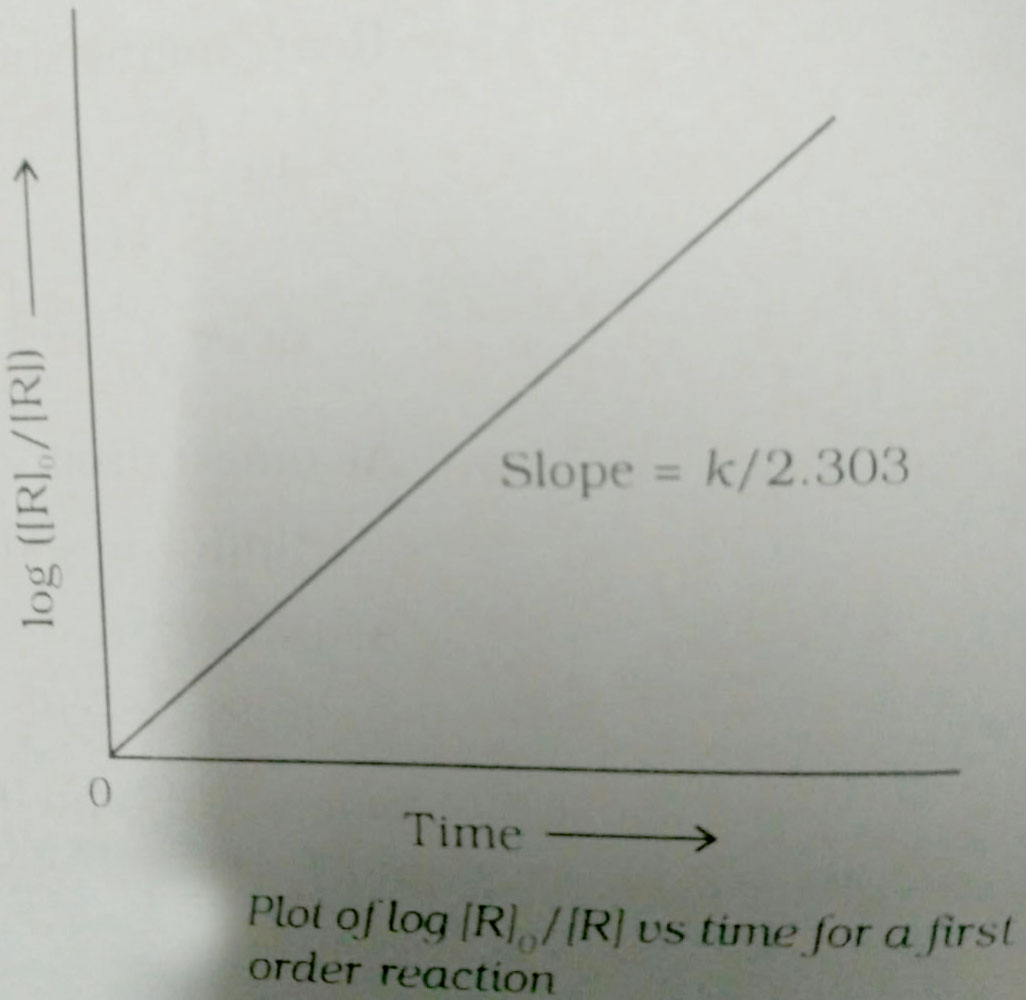Filters

Q&A - Ask Doubts and Get Answers
Q

Help me solve this The correct difference between first-second-order reactions is that

The correct difference between first-second-order reactions is that

• Option 1)

a first-order reaction can be catalyzed; a second-order reaction cannot be catalyzed

• Option 2)

the half-life of a first-order reaction does not depend on [A]o; the half-life of a second-order reaction does depend on [A]o

• Option 3)

the rate of a first-order reaction does not depend on reactant concentrations; the rate of a second-order reaction does depend on reactant concentrations

• Option 4)

the rate of a first-order reaction does depend on reactant concentrations; the rate of a second-order reaction does not depend on reactant concentrations

117 Views

As we learnt that

nth order reaction -

The rates of the reaction is proportional to nth power of reactant

- wherein

Differential rate law

Integrated rate laws,

a= initial, concentration of reactant at t=0 sec

x= concentration of product formed at t= tsec

First Order Reaction -

The rate of the reaction is proportional to the first power of the concentration of the reaction- wherein

Formula:

R            P

a                 0

a-x             x

[differentiate rate law]

Unit of

Option 1)

a first-order reaction can be catalyzed; a second-order reaction cannot be catalyzed

This is incorrect.

Option 2)

the half-life of a first-order reaction does not depend on [A]o; the half-life of a second-order reaction does depend on [A]o

This is correct.

Option 3)

the rate of a first-order reaction does not depend on reactant concentrations; the rate of a second-order reaction does depend on reactant concentrations

This is incorrect.

Option 4)

the rate of a first-order reaction does depend on reactant concentrations; the rate of a second-order reaction does not depend on reactant concentrations

This is incorrect.

Exams
Articles
Questions